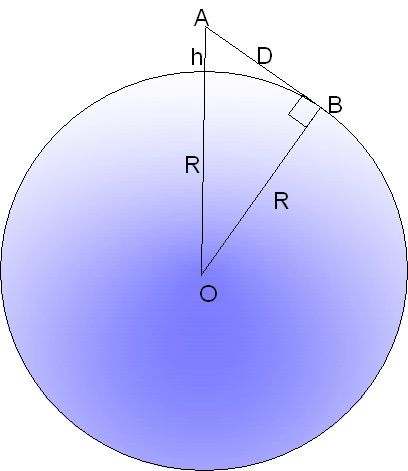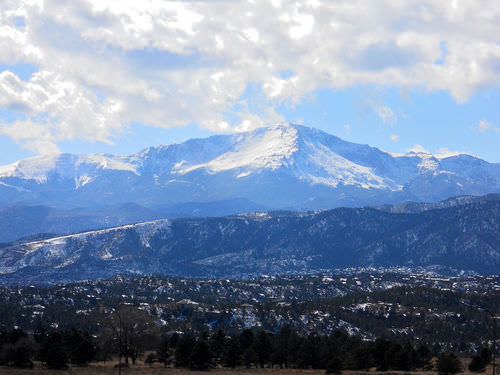# 17.1: Distance to the Horizon

•• Contributed by CK12
• CK12

Imagine you were standing at an elevation of $$h$$ meters above the ocean and looking out across the water. What is the distance $$D$$ to the horizon? It can be calculated, if you know the radius $$R$$ of the Earth.Figure $$\PageIndex{1}$$: Illustration of the horizon calculation.

Your line of sight to the horizon is a tangent to the Earth --- a line which touches the sphere of the Earth at just one point, marked $$B$$ in the drawing here. If $$O$$ is the center of the sphere of the Earth, by a well-known theorem of geometry such a tangent is perpendicular to the radius $$OB$$, that is, it makes a 90 angle with it. It follows from applying the Pythagorean theorem to $$\Delta AB$$ that:

$D^2\,+\,R^2\,=\,(R\,+\,h)^2$

$D^2\,=\,(R\,+\,h)^2\,-\,R^2$

$D^2\,=\,(R^2\,+\,2Rh)\,+\,h^2\,-\,R^2$

$D^2\,=\,2Rh\,+\,h^2$

$D^2\,=\,h(2R+h)$

Since the diameter $$2R$$ of the Earth is much bigger than h, we can replace ($$2R+h)$$ by $$2R$$ without significantly affecting the results. Carrying out this replacement gives:

$D^2\,=\,2Rh$

$D\,=\sqrt{2Rh}$

This equation lets one calculate $$D$$ in kilometers, if $$h$$ and $$R$$ are given in kilometers. We can also rewrite it as:

$D\,=\sqrt{2Rh}\,=\sqrt{2R}\times\sqrt{h}$

Using $$R\,=\,6371$$ km, we find

$D\,=\,112.88\,km\times\sqrt{h}$

If you are standing atop a mountain 1 km high, $$h=1$$ km and your horizon should by 112.88 km away (we neglect the refraction of light in the atmosphere, which may modify this value). From the top of Mauna Kea on Hawaii, an extinct volcano about 4 km high (also the site of important astronomical observations), the horizon should be about twice as distant, 226 km. On the other hand, standing on the beach with your eyes 2 meters = 0.002 km above the water, since $$\sqrt{0.002}\,=\,0.0442$$. the horizon is only 5 km away.

The calculation should also hold the other way around. From a boat on the ocean you should begin seeing the top of Mauna Kea after you pass a distance of 226 km (again, not accounting for refraction). On November 15, 1806, Lieutenant Zebulon Pike of the US Army, leading an exploration party across the plains of the midwestern US, saw through his spyglass the top of a distant peak, just above the horizon. It took his party a week to cover the 100 miles to the mountain, which is now known as Pike's Peak, one of the tallest in Colorado. Pike actually tried to climb to its top, but the snow and the unexpected height of the mountain forced him back.Figure $$\PageIndex{2}$$: Pike's Peak in Colorado.